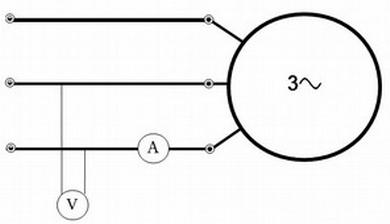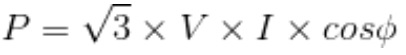# THREE PHASE ELECTRICAL CALCULATION

## Calculator

This tool performs the calculation for the power, voltage, intensity, and cos phi for any electrical three phase consumer.To use it, just select parameter to be calculated and fill the rest of fields:
 V (Line Voltage): V I (Intensity): A Cosφ (Cos Phi): P (Power): kW

The default option is a 3 phase power calculator, but any other parameter can be selected.

### Equation used for the calculation

The equation used for this calculation is:## Clarifications

### Power (P):

Absorbed electrical power. Please, note that this is not necessarily the nominal power of the equipment (maximum power) that usually refers to the mechanical power. For instance, the electrical power of a motor is the mechanical power at the shaft, and electrical power (P) will be always higher than shaft power because the efficiency of the motor is always below 100%

### Voltage (V):

This is the line voltage (measured between two phases).

### Intensity (I):

Current for any of the phases (assuming the system is in equilibrium, all phases have the same current).

### Cosine phi (Cosφ):

Relationship between active and apparent current. This value is provided by the equipment manufacturer for its nominal conditions. It is between 0 and 1. A very low Cosφ means that part of the intensity is not transformed in useful power. Some consumers, like electrical heaters, have Cosφ = 1.